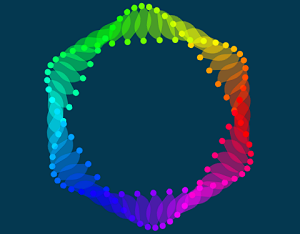Search IntMath
Close

450+ Math Lessons written by Math Professors and Teachers

5 Million+ Students Helped Each Year

1200+ Articles Written by Math Educators and Enthusiasts

Simplifying and Teaching Math for Over 23 Years

# CSS Animated Hexagon Wave

Page by Murray Bourne, IntMath.com. Last updated: 28 January 2019.

Most animations like the one below require javascript, but this one is pure CSS. It means it flows more smoothly than if we used a javascript approach, and takes less processing power.

## SCSS

This was my first experience working with SCSS ("Sassy CSS"), an extension language for CSS. The concept behind SCSS is you can write a .scss file using variables and loops, and a compiler will interpret that file into something the browser can use, and save it as a .css file (which you can seee the result of in the code for this page). See below the animation for more details.

## The Concept

There are 50 divs with class .hexagon, each containing a span.

The divs are translated by even amounts around the frame, and animated with a slight offset, giving the wave effect.

Tsankashvili's original Codepen made use of HAML to translate super-simple instructions into HTML, but I just coded the HTML directly (with the help of PHP).

## The SCSS

The SCSS makes use of these 3 variables:

$size: 50px;$circle-count: 50;
$speed: 3s; The variables are utilized in the following manner for the widths, heights and radii of the objects: .hexagon{ width:$size;
height: $size / 2; &, span{ border-top-left-radius:$size;
border-bottom-right-radius: $size; } ...  The coolest part is this loop, which sets the color, transform and animation properties of each of the divs: @for$i from 1 through $circle-count{ &:nth-child(#{$i}){
$color:$i * 360 / $circle-count; transform: rotate(-$i * 360deg / $circle-count) translate($size + 100px);
color: hsla($color,100%,50%,1); span{ animation-delay: -$i * $speed * 3 /$circle-count;
background-color: hsla(\$color,100%,50%,0.5);
}
}
}


For a compiler, I made use of Koala, a "GUI application for Less, Sass, Compass and CoffeeScript compilation", which was a 10-minute download and deploy process.

## The math

As usual with any animations involving circles and hexagone, trigonometry and geometry are involved, and of course transformational geometry as previously mentioned.

## Credits

Based heavily on Hexagon Codepen by Tsankashvili

### Screen shot

If you can't see the animation above, here's a screen shot:## Problem SolverThis tool combines the power of mathematical computation engine that excels at solving mathematical formulas with the power of GPT large language models to parse and generate natural language. This creates math problem solver thats more accurate than ChatGPT, more flexible than a calculator, and faster answers than a human tutor. Learn More.